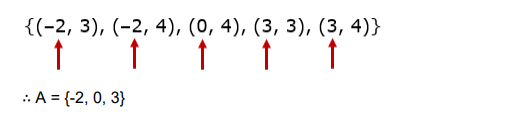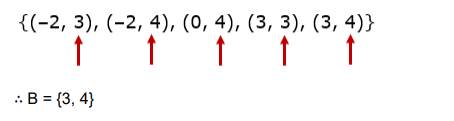# If A × B = {(–2, 3), (–2, 4), (0, 4), (3, 3), (3, 4), find A and B.

Question:

If $A \times B=\{(-2,3),(-2,4),(0,4),(3,3),(3,4)$, find $A$ and $B$.

Solution:

Here, A × B = {(–2, 3), (–2, 4), (0, 4), (3, 3), (3, 4)} To find: A and B

Clearly, A is the set of all first entries in ordered pairs in A × Band B is the set of all second entries in ordered pairs in A × B